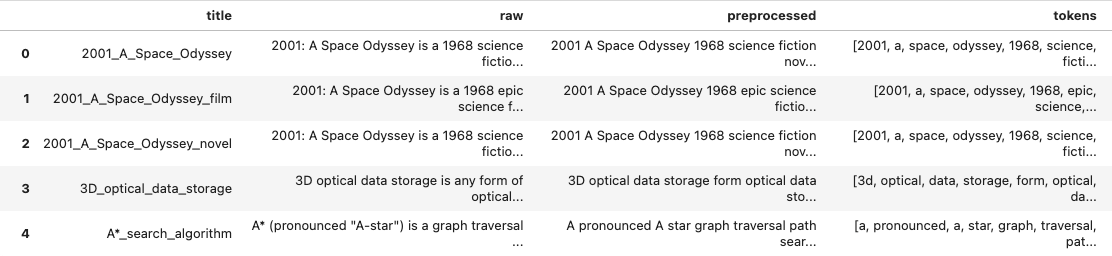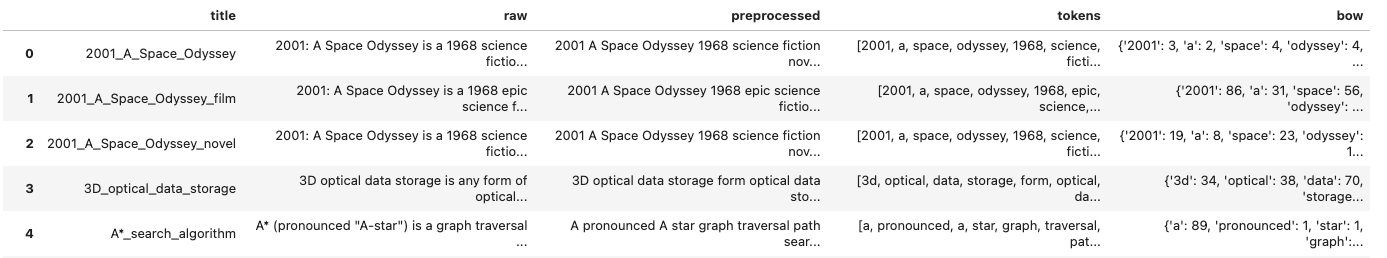# NLP: Text Vectorization Methods with SciKit Learn

Posted in Nlp, Python, Word_vectors, Scikit_learn

SciKit Learn is an extensive library for machine learning projects, including several classifier and classifications algorithms, methods for training and metrics collection, and for preprocessing input data. In every NLP project, text needs to be vectorized in order to be processed by machine learning algorithms. Vectorization methods are one-hot encoding, counter encoding, frequency encoding, and word vector or word embeddings. Several of these methods are available in SciKit Learn as well.

This article is an in-depth explanation and tutorial to use all of SciKit Learns preprocessing methods for generating numerical representation of texts. For each of the following vectorizers, a short definition and practical example will be given: one-hot, count, dict, TfIdf and hashing vectorizer.

The technical context of this article is `Python v3.11` and `scikit-learn v1.2.2`. All examples should work with newer versions too.

## Requirements and Used Python Libraries

This article is part of a blog series about NLP with Python. In my previous articles, I covered how to create a Wikipedia article crawler, which will take an article name as the input, and then systematically downloads all linked articles until the given total number of articles or depth is achieved. The crawler produces text files, which are then further processed by an `WikipediaCorpus` object, a self-created abstraction on top of NLTK, that gives convenient access to individual corpus files and corpus statistics like number of sentences, paragraphs and the vocabulary. In this article, the `WikipediaCorpus` object is merely used to load specific articles that serve as examples for applying the Sci-Kit learn preprocessing methods.

## Basic Example

The following snippets shows how to use the `WikipediaCorpus` object to crawl 200 pages starting with machine learning and artificial intelligence.

``````reader = WikipediaReader(dir = "articles3")
``````

On top of this, the corpus object is created:

``````corpus = WikipediaPlaintextCorpus('articles3')
corpus.describe()
#{'files': 189, 'paras': 15039, 'sents': 36497, 'words': 725081, 'vocab': 32633, 'max_words': 16053, 'time': 4.919918775558472}
``````

Finally a SciKit Learn pipeline is created that will preprocess the text (ignore all non-text characters and stop words) and tokenized.

``````pipeline = Pipeline([
('corpus', WikipediaCorpusTransformer(root_path='./articles3')),
('preprocess', TextPreprocessor(root_path='./articles3')),
('tokenizer', TextTokenizer()),
])
``````

This results in the following data frame on which the following sections apply further processing steps:## One-Hot Vectorizer

A one-hot-encoding is a sparse matrix in which the rows represent documents, and the columns represent words from the vocabulary. Each cell has a value of either 0 or 1 to indicate if a word occurs in the document.

SciKit learns `OneHotEncoder` was one of the hardest to get working with data contained in the Pandas data frame object, because it is designed to work with ordinal data represented as tuples. Therefore, the Pandas series object containing the preprocessed tokens need to be transformed to a matrix first, with the special challenge to pad and trim the number of columns to a reasonable value. The helper methods is as follows:

``````def to_matrix(series, padding_value):
matrix = []
for vec in np.nditer(series.values,flags=("refs_ok",)):
v = vec.tolist()

return matrix
``````

Here is an example of the resulting data structure, including padding, when applied to the artificial intelligence article:

``````x_t = to_matrix(X['tokens'], 10000)
#['a.i', '.', 'artificial', 'intelligence', 'simply', 'a.i', '.', '2001', 'american', 'science', #'fiction', 'film', 'directed', 'steven', 'spielberg', '.', 'the', 'screenplay', 'spielberg', #'screen', 'story', 'ian', 'watson', 'based', '1969', 'short', 'story', 'supertoys', 'last',
# ... '', ''
``````

Following example shows how to apply the one-hot-encode to the padded token list:

``````from sklearn.preprocessing import OneHotEncoder

vectorizer = OneHotEncoder(handle_unknown='ignore')
x_train = vectorizer.fit_transform(np.array(x_t).reshape(-1, 1))

print(type(x_train))
#scipy.sparse._csr.csr_matrix

print(x_train)
#  (0, 7) 1.0
#  (1, 8) 1.0
#  (2, 87) 1.0
#  (3, 64) 1.0
#  (4, 5) 1.0
#  (5, 80) 1.0
#  (6, 42) 1.0

print(vectorizer.get_feature_names_out())
# ['x0_,' 'x0_.' 'x0_...' 'x0_1948' 'x0_1953' 'x0_1968' 'x0_1972' 'x0_2001'
# 'x0_a' 'x0_additionally' 'x0_after' 'x0_amazing' 'x0_arthur' 'x0_artwork'
# 'x0_background' 'x0_based' 'x0_bbc' 'x0_before' 'x0_both' 'x0_breaking'
# 'x0_c.' 'x0_carnon' 'x0_clarke' 'x0_collaboration' 'x0_comet' 'x0_comics'

print(len(vectorizer.get_feature_names_out()))
# 34853
``````

The encoder produced `34853` features and has feature names for decoding purposes.

## Count Vectorizer

The count vectorizer is a customizable SciKit Learn preprocessor method. It works with any text out of the box, and applies preprocessing, tokenization and stop words removal on its own. These tasks can be customized, for example by providing a different tokenization method or stop word list. (This applies to all other preprocessors as well.) Applying the count vectorizer to raw text creates a matrix in the form of `(document_id, tokens)` in which the values are the token count.

This vectorizing method works without any configuration and without any additional transformations directly on the preprocessed text:

``````from sklearn.feature_extraction.text import CountVectorizer

vectorizer = CountVectorizer()
x_train = vectorizer.fit_transform(X['preprocessed'])
``````

Its application is shown in the following snippet:

``````print(type(x_train))
#scipy.sparse._csr.csr_matrix

print(x_train.toarray())
# [[0 0 0 ... 0 0 0]
# [0 7 0 ... 0 0 0]
# [0 3 0 ... 0 0 0]
# ...
# [0 0 0 ... 0 0 0]
# [0 1 0 ... 0 0 0]
# [0 2 0 ... 0 0 0]]

print(vectorizer.get_feature_names_out())
# array(['00', '000', '0001', ..., 'zy', 'zygomaticus', 'zygote'],
#      dtype=object)

print(len(vectorizer.get_feature_names_out()))
# 32417
``````

This vectorizer detects `32417` features and also has decodable feature names.

## Dict Vectorizer

As the name of this vectorizer implies, it turns dictionary objects containing texts into a vector. Therefore, it cannot work in raw text, but requires a frequency-encoded representation of the text, for example a bag-of-word representation.

The following helper method provides a bag-of-words representation from the tokenized texts.

``````from collections import Counter

def bag_of_words(tokens):
return Counter(tokens)

X['bow'] = X['tokens'].apply(lambda tokens: bag_of_words(tokens))
``````

The resulting dataframe looks as follows:And the bow for the machine learning article is this:

``````X['bow']
# Counter({',': 418,
#  '.': 314,
#  'learning': 224,
#  'machine': 124,
#  'data': 102,
#  'training': 49,
#  'algorithms': 46,
#  'model': 38,
#  'used': 35,
#  'set': 31,
#  'in': 30,
#  'artificial': 29,
``````

The following code shows how to apply the vectorizer to the additionally created bag-of-words representation.

``````from sklearn.feature_extraction import DictVectorizer

vectorizer = DictVectorizer(sparse=False)
x_train = vectorizer.fit_transform(X['bow'])

print(type(x_train))
#numpy.ndarray

print(x_train)
#[[ 15.   0.  10. ...   0.   0.   0.]
# [662.   0. 430. ...   0.   0.   0.]
# [316.   0. 143. ...   0.   0.   0.]
# ...
# [319.   0. 217. ...   0.   0.   0.]
# [158.   0. 147. ...   0.   0.   0.]
# [328.   0. 279. ...   0.   0.   0.]]

print(vectorizer.get_feature_names_out())
# array([',', ',1', '.', ..., 'zy', 'zygomaticus', 'zygote'], dtype=object)

print(len(vectorizer.get_feature_names_out()))
# 34872
``````

This vectorizer detects `# 34872` features and also has decodable feature names.

## TfIdf Vectorizer

The Term Frequency/Inverse Document Frequency is a well-known metric in information retrieval. It encodes word frequencies in such a way as to put equal weight to common terms that occur in many documents, as well as uncommon terms only present in a few documents. This metric generalizes well over large corpora and improves finding relevant topics.

The SciKit Learn implementation is straight forward to use on the already preprocessed text. But keep in mind that its internal steps like preprocessing and tokenization can be customized as required.

Creating this vectorizer is simple:

``````from sklearn.feature_extraction.text import TfidfVectorizer

vectorizer = TfidfVectorizer()
x_train = vectorizer.fit_transform(X['preprocessed'])
``````

And here are the results of applying the vectorizer to the corpus.

``````print(type(x_train))
# scipy.sparse._csr.csr_matrix

print(x_train.shape)
# (189, 32417)

print(x_train)
#  (0, 9744) 0.09089003669895346
#  (0, 24450) 0.0170691375156829
#  (0, 6994) 0.07291108016392849
#  (0, 6879) 0.05022416293169798
#  (0, 10405) 0.08425453878004373
#  (0, 716) 0.048914251894790796
#  (0, 32066) 0.06299157591377592
#  (0, 17926) 0.042703846745948786

print(vectorizer.get_feature_names_out())
# array(['00' '000' '0001' ... 'zy' 'zygomaticus' 'zygote'], dtype=object)

print(len(vectorizer.get_feature_names_out()))
# 32417
``````

This vectorizer detects `32417` features and also has decodable feature names.

## Hashing Vectorizer

The final preprocessor is at the same time the most generic and the most performant. It creates a structured matrix with `document_id x tokens`, but the values can be normalized as L1 (sum of its values) or L2 (square root of the sum of all squared vector values). It has further benefits such as requiring low memory and being suitable to process streamed documents.

The HashingVector works as-is on the preprocessed text.

``````from sklearn.feature_extraction.text import HashingVectorizer

vectorizer = HashingVectorizer(norm='l1')
x_train = vectorizer.fit_transform(X['preprocessed'])
``````

And it produces the following results:

``````print(type(x_train))
#scipy.sparse._csr.csr_matrix

print(x_train.shape)
#(189, 1048576)

print(x_train)
# (0, 11631) -0.006666666666666667
#  (0, 17471) -0.006666666666666667
#  (0, 26634) 0.006666666666666667
#  (0, 32006) -0.006666666666666667
#  (0, 42855) 0.006666666666666667
#  (0, 118432) 0.02666666666666667
#  (0, 118637) -0.006666666666666667

print(vectorizer.get_params())
#{'alternate_sign': True, 'analyzer': 'word', 'binary': False, 'decode_error': 'strict', 'dtype': <class 'numpy.float64'>, 'encoding': 'utf-8', 'input': 'content', 'lowercase': True, 'n_features': 1048576, 'ngram_range': (1, 1), 'norm': 'l1', 'preprocessor': None, 'stop_words': None, 'strip_accents': None, 'token_pattern': '(?u)\\b\\w\\w+\\b', 'tokenizer': None}
``````

This vectorizer detects `1048576` features in the documents. Alas, its feature names can not be decoded.

## Conclusion

This article is an in-depth tutorial to SciKit learn built-in text vectorization methods. For each of the following vectorizer, you saw a practical example and how to apply them to text: one-hot, count, dictionary, TfIdf, hashing. All vectorizers work out-of-the box with default text processing utilities, but you can customize the preprocessor, tokenizer and top words. Also, they can work with raw text itself, except the dictionary, which requires a bag-of-word representation of text, and the one-hot encoder, which requires a manual conversion from a Pandas series to Numpy matrix with token padding.